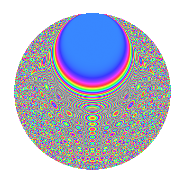# Properties

 Label 1050.2.bkLevel 1050 Weight 2 Character orbit bk Rep. character $$\chi_{1050}(113,\cdot)$$ Character field $$\Q(\zeta_{20})$$ Dimension 480 Sturm bound 480

# Related objects

## Defining parameters

 Level: $$N$$ = $$1050 = 2 \cdot 3 \cdot 5^{2} \cdot 7$$ Weight: $$k$$ = $$2$$ Character orbit: $$[\chi]$$ = 1050.bk (of order $$20$$ and degree $$8$$) Character conductor: $$\operatorname{cond}(\chi)$$ = $$75$$ Character field: $$\Q(\zeta_{20})$$ Sturm bound: $$480$$

## Dimensions

The following table gives the dimensions of various subspaces of $$M_{2}(1050, [\chi])$$.

Total New Old
Modular forms 1984 480 1504
Cusp forms 1856 480 1376
Eisenstein series 128 0 128

## Trace form

 $$480q - 8q^{3} + O(q^{10})$$ $$480q - 8q^{3} + 8q^{12} - 8q^{15} + 120q^{16} + 8q^{18} + 80q^{19} + 32q^{22} + 200q^{25} - 8q^{27} + 32q^{33} + 80q^{34} + 40q^{37} + 40q^{39} + 16q^{40} - 16q^{43} + 40q^{45} + 8q^{48} + 32q^{55} - 32q^{57} + 16q^{58} - 40q^{60} + 32q^{63} - 160q^{67} - 8q^{70} - 8q^{72} - 112q^{73} - 32q^{75} - 192q^{78} - 160q^{79} + 80q^{81} - 64q^{82} - 8q^{85} - 120q^{87} - 8q^{88} - 40q^{90} - 96q^{93} - 16q^{97} + O(q^{100})$$

## Decomposition of $$S_{2}^{\mathrm{new}}(1050, [\chi])$$ into newform subspaces

The newforms in this space have not yet been added to the LMFDB.

## Decomposition of $$S_{2}^{\mathrm{old}}(1050, [\chi])$$ into lower level spaces

$$S_{2}^{\mathrm{old}}(1050, [\chi]) \cong$$ $$S_{2}^{\mathrm{new}}(75, [\chi])$$$$^{\oplus 4}$$$$\oplus$$$$S_{2}^{\mathrm{new}}(150, [\chi])$$$$^{\oplus 2}$$$$\oplus$$$$S_{2}^{\mathrm{new}}(525, [\chi])$$$$^{\oplus 2}$$

## Hecke Characteristic Polynomials

There are no characteristic polynomials of Hecke operators in the database